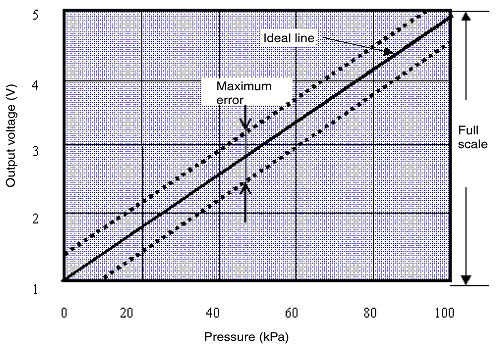# What is linear accuracy of Pressure Sensors?

Linear accuracy, expressed as a percentage of full scale, is the amount of deviation from the rated values for output current (4 mA and 20 mA) or voltage (1 V and 5 V) when zero pressure and the rated pressure are applied at a constant temperature of 23°C.Example: The accuracy for E8M-10 Pressure Sensor is ±5% FS.

Converting this into voltage,

±5% × 1⁄1000 (%) × 4 V (FS) = ±0.2 V,

so the line will deviate ±0.2 V max.